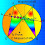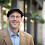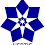## Sunday, May 18, 2008

### Elearn Geometry Problem 6See complete Problem 6 at:
www.gogeometry.com/problem/problem006.htm

Triangle, cevian, equal segments, and angles. Level: High School, SAT Prep, College geometry

1.CD/BD = sin(6x)/sin(3x)= 2cos(3x)
AB/BD = sin(3x)/sin(2x)
Thus, 2cos(3x) = sin(3x)/sin(2x)
or sin(2x) = (1/2)* sin(3x)/cos(3x)
Now if x=15 then sin(2x)=1/2 & sin(3x)=cos(3x) which satisfies the equation.
So x = 15 deg.
Ajit: ajitathle@gmail.com

2.http://ahmetelmas.files.wordpress.com/2010/05/cozumlu-ornekler.pdf

3.bae deok rak(bdr@korea.com)October 3, 2010 at 2:10 AM

Let E be a point with triangles DBA and EDC are congruence(AB=CD,AD=CE,ang(BAD)=x=ang(DCE), ang(ACE)=4x). Then DB=DE and ang(EDC)=2x and hence
ang(EBD)=x=ang(BED).
Furthermore, since BA=CD, BE=EC=AD=CA and ang(EBA)=3x=ang(ACD), triangles BAE and CDA are congruence. we see that AE=AD and so triangle AEC is an equilateral. we get 4x=60 degree, that is, x=15 degree.

1.In case anyone got confused, he swapped A and B. Replace every A with B and vice versa and it should become clear what he meant.
Also, point E lays below DC in his solution, closer to the D point (at first there are four different orientations for this construction).

4.Comment on "Joe": Trial-error is not accepted in the Netherlands

Comment on "bae deaok rak":1)Where did you put point E? 2)AD=CA is not true

5.Given:
ABC=180
A=2x
B=x+90(90 because that is right angle)
C=3x
Solution:
ABC=A+B+c
180=2x+x+90+3x
180=6x+90
-6x=90-180
-6x/-6=-90/-6
x=15

x=15+90 =2x =3x
=2(15) =3(15)
=30 =45
180=15+90+30+45
180=180

1.El enunciado nunca dice que sea un angulo recto, hay que demostrarlo

6.To Jonathan (Problem 6): In your statement B=x+90.... 90 is not a data.

7.if you notice the DBC this is 90%. and why B=x+90 because that is seperated. i'm right??

8.Video solution (Spanish version) by Eder Contreras and Cristian Baeza at Geometry problem 6. Thanks Eder and Cristian.

9.10.Greetings.
In your solution of problem 6, there’s something I didn’t understand.
The first circle is defined as having center at the midpoint G of AB (with diameter AB) and the second circle has center at the midpoint E of CD (with diameter CD).
I can’t see why the first circle meets CD in its midpoint E. If we don’t assure that E lies on the first circle, it’s not clear that AG = GE.

11.To Nilton Lapa
Greetings to you too.
Did you notice DBC is an Isosceles triangle.
Since E is the Mid point of DC BEC is 90 Deg.
As AB is the diameter of the first circle according to the angle on semi circle is 90 Deg
The first circle should go thriugh E.

Thanks.
..............

1.Another way to clear up this confusion about the first circle passing through E:

∠EDB is exterior of △ADB, so ∠EDB = ∠ABD + ∠DAB = x + 2x = 3x.
Therefore △DBC is isosceles (same base angles, ∠EDB = 3x = ∠BCE), that is, BD = BC.
Drop a perpendicular from B to DC (that is, BE⟂DC). As it is the altitude in an isosceles △DBC, it divides its base into equal pieces, so ED = EC and E is a midpoint of DC.

Now the part regarding the circle:
Note that △ABE is a right triangle, since BE⟂DC (and also BE⟂AC, because A,D,C are collinear).
Since we've chosen G to be the midpoint of its hypotenuse AB (AG = GB), the segment GE is a median in a right triangle △ABE, so it must also be equal to these parts: GA = GB = GE (three radii of the first circle).
Therefore points A, B, E lie on the same circle centered at G.

Rengaraj's response assumes that E lies on the circle when it says that it is a right triangle in a circle.

12.Thanks. The explanation is absolutly clear. My doubt in problem 6 was enlighted.
Nilton Lapa

13.E es el punto sobre D-C tal que AD=DE
F es tal que B-F paralelo a A-C y BF=AD
D-B es paralela a E-F
G es intersección entre E-F y B-C formando EBFC cíclico
ang(DBC) = 180°-6α
△FCD es isósceles
(1) ang(FCD) =ang(DFC)=90°-α
△BGE es congruente a △FGC por la simetría
general en los triángulos isósceles △BFE y △EGC
y el paralelismo de BF con EC.
ang(CEB) = 90°-α
ang(EBF)=ang(BFC)=[360-2(90°-α)]/2=90°+α
ang(FHB)=180°-(90°+α)-2α=90°-3α
ang(EHF)=3α
Entonces △ABD es semejante a △EFH, por lo tanto
(2) ang(GEB)=ang(FCG)=2α
ang(FCE)=90°-α=ang(FCG)+ang(BCA)=5α
6α=90°; α=15° Q.E.D.

14.Let E be a point on the extension AB such that AE=AC.
Bisect ∠A and let a point F on the angle bisector of ∠A such that m∠AEF=m∠ACF=x.
Then ∆AFE≅∆AFC by ASA and at the same time they are both isosceles.
Let a point H on BC such that the angle bisector of ∠A intersect BC at H.
Then m∠CFH=m∠EFH=m∠FEH=m∠FCG=2x, also m∠EBC=5x and m∠EGC=6x. An exterior angle is equal to the sum of its remote interior angles.
Again by ASA, ∆EHF≅∆CHF and they are also isosceles triangles, then EH=FH=CH.
By perpendicular bisector concurrence theorem, CG⊥EF that makes ∠EGC a right angle.
Therefore: 6x=90°,x=15°

15.What is G

1.Couldn't find the G spot, eh? ;)
Judging by the later statement about "CG⊥EF", I would say it must be the intersection point of EF and CB (just draw it in proportion and knowing the end result, and you'll see).

Still I don't quite follow the end of the proof, about using the "perpendicular bisector concurrence theorem", since nowhere has been proved that CH and EH are perpendicular bisectors of CF and EF (even if I can see it in the picture). The train of thoughts might be correct, but the proof is still incomplete.

16.See my proof, maybe similar to the video-one mentioned by the owner of the site on Sept, 4, 2011, but I am not able to see that.

1.To Stan Fulger
Spanish solution video at http://www.gogeometry.com/geometria/p006_solucion_problema_video_triangulo_angulo_ceviana_trazo_auxiliar.htm
Thanks

17.I think this is different from the previous solutions.

18.19.There are so many solutions, but I think this is a new one
ang(BDC)=3x
Drop perpendicular BE on AC ; M on AB with MA=MB=> AM=BM=ME=EC=DE=a
From ME=AM => ang(AEM)=2x ; from ME=EC => ang(EMC)=ang(ECM)=x => ang(MCB)=2x
triangle ABC and CBM are similar => BC/MB=AB/BC +> BC^2 = 2a^2 => BC = a sqrt(2)
In triangle EBC is BE=EC => triangle MEB is equilateral => ang(ABE)=60 => 2x=30 => x=15

1.It's the same solution as the Turkish one.

20.angle BDC =angle BCD =3x;
make E midpoint of DC, BE perpendicular to DC;
Make K midpoint of AB;
connect KE;
so AK=KB=KE =DE=EC;
because KE=DE=EC' so triangle DKC is right triangle;
because AK=KE; so angle KEA=angle KAE = 2x;
so angle ECK =x;
so DKBC on the same circle,
so angle DBC =angle DKC =90;
so 6x =90, x=15;

21.1.In your solution I don't see any proof that the second circle indeed passes through B. Don't depend on GeoGebra's drawings, they can be deceiving; depend on math & logic. Wenlong's solution above contains the extra step needed to prove that DKBC (your DFBC) is cyclic and B indeed lies on the same circle with D, F and C.

22.B belongs to the first circle (center F)
<FBD =x intercepts the second circle (center E) on arc FD
<FEB=2x intercepts the second circle (center E) on arc FD
This is only possible if B belongs to the second circle
Therefore B belongs to the two circles

23.Let E be the mid point of CD and let F be the midpoint of AB

So AF = FB = FE = DE = CE = c/2

< AEX = 2x and < EFC = < ECF = x, so < BCF = 2x

Hence BC^2 = a^2 = BF.AB = c^2/2 = BE^2 + CE^2 = BE^2 + c^2/4
Therefore BE = c/2 = CE and so < BCE = 3x = 45 and x = 15

Sumith Peiris
Moratuwa
Sri Lanka

24.Grab a pen and a piece of paper, and illustrate along.

Before we begin, let us see what new info we can obtain:
> Angle ABC = 180° - 2x - 3x = 180° - 5x
> Angle ADB = 180° - x - 2x = 180° - 3x
> Angle BDC = 180° - (180° - 3x) = 3x
> Since angles BDC and BCD are equal, triangle BCD is isosceles.
> Since triangle BCD is isosceles, line BD = line BC

Add point E to the figure and connect it to points C and D such that a duplicate of triangle ABD is formed inside triangle BCD. Do note that point E should be closer to point D than point C, or else the solution would not work.

At this point, we can see that:
Since triangles ABD and CDE are congruent.
· Angle CDE = 2x
· Angle ACE = x
· Line AD = line DE
· Line CE = line BC = line BD
> Angle BDE = 3x - 2x = x
> Angle ADE = 180° - 3x + x = 180 - 2x

Now connect point E to point A. After this point we shall use the same solution as we did in problems 4 and 5.

Notice that:
> Angle CAE = (180° - (180° - 2x))/2 = 2x/2 = x
> Triangle ACE is isosceles as angles CAE and ACE are equal.
> Line AE = line CE because triangle ACE is isosceles.

Add point F on line AB and connect it to point C such that CF is the angle bisector of angle BCE. Lastly, connect point F to point E.

We can see:
> Angle ACF = x + x = 2x = Angle CAF
> Triangle ACF is isosceles because angles ACF and CAF are equal.
> Line AF = line CF since triangle ACF is isosceles.
> Triangles AEF and CEF are congruent by SSS because:
· Line AE = line CE
· Line AF = line CF
· They share side EF.
> Triangles CEF and CBF are congruent by SAS because:
· Line CE = line BC
· Angle ECF = angle BCF = x
· They share side CF
> Since triangles AEF, CEF and CBF are congruent:
· Angles AFE, CFE and CFB are equal.

Now, we solve the problem:
> Angle AFE + angle CFE + angle CFB = 3(Angle CFB) = 180°
> Angle CFB = 180°/3 = 60°
> Since all 3 angles in a triangle add up to 180°,
x + 180° - 5x + 60° = 180°
240° - 4x = 180°
4x = 60°
x = 60°/4 = 15°

25.Considering trinagle BCD
sin3x/BD=sin6x/CD
CD=BDsin6x/sin3x

Considering triangle ABC
sin2x/BC=sin3x/AB
AB=BCsin3x/sin2x

Since CD=AB & BD=BC
sin6x/sin3x=sin3x/sin2x
2cos3x=sin3x/sin2x
2sin2xcos3x=sin3x
sin5x-sinx=sin3x
sin5x-sin3x=sinx
2cos4xsinx=sinx
cos4x=1/2 or sinx=0(rej.)
4x=60
x=15